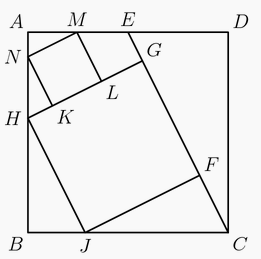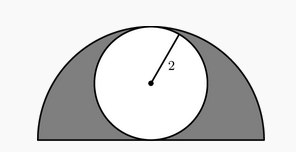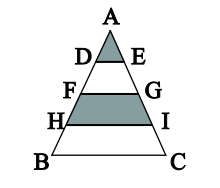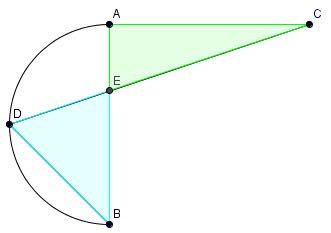###### back to index | new

In the diagram below, $ABCD$ is a square. Point $E$ is the midpoint of $\overline{AD}$. Points $F$ and $G$ lie on $\overline{CE}$, and $H$ and $J$ lie on $\overline{AB}$ and $\overline{BC}$, respectively, so that $FGHJ$ is a square. Points $K$ and $L$ lie on $\overline{GH}$, and $M$ and $N$ lie on $\overline{AD}$ and $\overline{AB}$, respectively, so that $KLMN$ is a square. The area of $KLMN$ is $99$. Find the area of $FGHJ$.Triangle $ABC$ has vertices $A = (3,0)$, $B = (0,3)$, and $C$, where $C$ is on the line $x + y = 7$. What is the area of $\triangle ABC$?

A circle of radius $2$ is inscribed in a semicircle, as shown. The area inside the semicircle but outside the circle is shaded. What fraction of the semicircle's area is shaded?In $\triangle{ABC}$, segments AB and AC have each been divided into four congruent segments. We must find the fraction of the triangle that is shaded.In $\bigtriangleup ABC$, $AB=BC=29$, and $AC=42$. What is the area of $\bigtriangleup ABC$?

In the diagram, AB is the diameter of a semicircle, D is the midpoint of the semicircle, angle $BAC$ is a right angle, $AC=AB$, and $E$ is the intersection of $AB$ and $CD$. Find the ratio between the areas of the two shaded regions.What is Heron's formula to calculate a triangle's area given the lengths of three sides?

Let $ABCD$ be a convex quadrilateral and the two diagonals intersect at point $O$. If $AC = 12, BD=5$ and $\angle{AOB}=2\angle{BOC}$, find the area of $S_{ABCD}$